Published: 15 May 2017

# Vibrational method of cleaning of surfaces from homogeneous waste materials

K. Ragulskis1
A. Bubulis2
D. Mažeika3
R. Kandrotaitė-Janutienė4
L. Ragulskis5
A. Bartkus6
1Lithuanian Academy of Sciences, Gedimino str. 3, 02189, Vilnius, Lithuania
2Kaunas University of Technology, Mechatronics Institute, Studentu str. 56, 51424, Kaunas, Lithuania
3, 4, 6Kaunas University of Technology, Department of Production Engineering, Studentu 56, 51424, Kaunas, Lithuania
5Vytautas Magnus University, K. Donelaičio str. 58, 44248, Kaunas, Lithuania
Corresponding Author:
A. Bubulis
Views 232

#### Abstract

Cleaning of contaminated surfaces is closely related to the study of adhesion forces between particles and the surface. Despite numerous technological advancements, the adhesion of particles and surfaces is a phenomenon that is far from being fully understood. This is due to many factors acting during process, - such as the surface roughness and material type, size, shape, electrostatic properties of particles, etc. A surface cleaning device based on nonlinear vibration of the contact element is presented in this paper. Relationships describing the periodic motion in steady state operating regimes are obtained. Characteristics of motion as functions of the frequency of excitation are investigated. Experimental investigations were performed for the determination of the influence of the cleaning velocity on the vibration frequency. It is shown that this dependence is directly related to adhesion forces between particles and the surface. Test results are in good accordance with theoretical predictions.

## 1. Introduction

Various cleaning techniques are used with particle contamination. Wet cleaning with chemical solution was the most widely used in the semiconductor industry. It has many advantages for particle cleaning but some problems such as high maintenance cost, large footprint, difficulty in equipment clustering, big environmental load, etc. can be outlined. Various physical cleaning methods capable of removing nanoscale contaminants such as megasonic cleaning, cryogenic cleaning, high-pressure jet cleaning and other methods were developed in order to overcome these problems . However, they are difficult to apply for nanoscale particle removal. In nanoscale cleaning, the particles are difficult to remove from the surface due to the relationship between the adhesion and cleaning forces. Thus, improving the cleaning performance the application of physical cleaning methods is required.

Non-chemical submerged surface cleaning is accomplished through the application of different methods like laser cleaning , the methods of cavitation bubble dynamics  or with solid CO2 (dry ice blasting)  with the the purpose to lift adhered particles out of the surface. This can be achieved by using low frequency vibrations, as well [5, 6].

A Model of the surface of the system which is contaminated by a homogeneous layer is investigated as a system with concentrated parameters (Fig. 1 and Fig. 2).

The system is described by the following differential equation:

1
$m\frac{{d}^{2}x}{d{t}^{2}}+{F}_{f}\left(\frac{dx}{dt}\right)+F\left(x\right)={P}_{0}+{P}_{1}\mathrm{c}\mathrm{o}\mathrm{s}\omega t,$

where $m$ is the reduced mass of the investigated system, $x$ is the displacement, $t$ is the time variable, ${F}_{f}\left(\frac{dx}{dt}\right)$ is the force of adhesion of the interaction of waste material with the surface which is being cleaned, $F\left(x\right)$ is the elastic dissipative force, ${P}_{0}+{P}_{1}\mathrm{c}\mathrm{o}\mathrm{s}\omega t$ is the external force acting to the mass $m$, ${P}_{0}$ is the constant applied force, ${P}_{1}$ is the amplitude of harmonic excitation, $\omega$ is the angular frequency of harmonic excitation.

Fig. 1Model of the system: 1 – surface of the body which is being cleaned, 2 – waste material, 3 – reduced mass, onto which the external excitation is acting, 4 – schematic representation of the elastic dissipative element with the hysteresis loop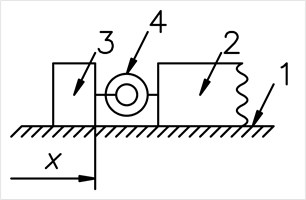Fig. 2Diagram of operation of the elastic dissipative element with the hysteresis loop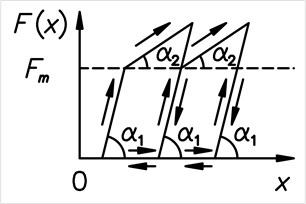On the basis of Fig. 2 the following relationships hold:

2
$\mathrm{t}\mathrm{a}\mathrm{n}{\alpha }_{1}={C}_{1},$
3
$\mathrm{t}\mathrm{a}\mathrm{n}{\alpha }_{2}={C}_{2},$

where ${\alpha }_{1}$ and ${\alpha }_{2}$ are the angles of the lines with $x$ coordinate axis of $s$.

The force of adhesion is of the following form:

4
${F}_{f}\left(\frac{dx}{dt}\right)={H}_{0}\mathrm{s}\mathrm{g}\mathrm{n}\frac{dx}{dt}+{H}_{1}\frac{dx}{dt},$

where ${H}_{0}$ is the coefficient of dry friction, ${H}_{1}$ is the coefficient of viscous friction.

When performing numerical calculations dry friction is assumed as an approximation shown in Fig. 3.

Fig. 3Approximation of dry friction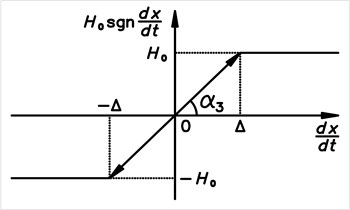On the basis of Fig. 3 the following relationship holds:

5
$\mathrm{t}\mathrm{a}\mathrm{n}{\alpha }_{3}=\frac{{H}_{0}}{\mathrm{\Delta }},$

where ${\alpha }_{3}$ is the angle of the line with x coordinate axis of s and $\mathrm{\Delta }$ defines the transitional region of supplementary viscous friction.

Vibrations of a device described by a nonlinear differential equation are investigated. Graphical relationships describing motion in the steady state regimes are obtained. Characteristics of motion as functions of frequency of excitation are investigated.

Numerical investigations performed in this paper are based on the material described in [1, 2].

The function $F$ is calculated as:

6
$F={F}_{p}+C\left(x-{x}_{p}\right),$

where the subscript $p$ indicates the quantity in the previous moment of time and when the following conditions hold:

7
$F\le 0,$

and:

8
$C>0.$

The value of $x$ is assigned to $\stackrel{-}{x}$ and then the following calculation is performed:

9
$C=\left\{\begin{array}{ll}{C}_{2},& F\ge {F}_{m},\mathrm{}\mathrm{}\mathrm{}\frac{dx}{dt}\ge 0,\\ 0,& F\le 0,\mathrm{}\mathrm{}\mathrm{}x\le \stackrel{-}{x},\\ {C}_{1},& \text{elsewhere},\end{array}\right\$

where ${C}_{1}$ and ${C}_{2}$ are coefficients of stiffness, ${F}_{m}$ is the limit of elastic behavior.

The function $H$ is defined as an approximation to dry friction ${H}_{0}\mathrm{s}\mathrm{g}\mathrm{n}\frac{dx}{dt}$ and is calculated as:

10
$H={H}_{p}+\stackrel{-}{C}\left[\frac{dx}{dt}-{\left(\frac{dx}{dt}\right)}_{p}\right],$

where:

11
$\stackrel{-}{C}=\left\{\begin{array}{ll}0,& \left|\frac{dx}{dt}\right|\ge \mathrm{\Delta },\\ \frac{{H}_{0}}{\mathrm{\Delta }},& \text{elsewhere}.\end{array}\right\$

Characteristics of steady state motion of the system:

Average power of the forces of dissipation:

12
${P}_{d}=\frac{\int \left({H}_{1}\frac{dx}{dt}+H+F\right)\frac{dx}{dt}dt}{\frac{2\pi }{\omega }}.$

Average power of the forces of excitation:

13
${P}_{F}=\frac{\int \left({P}_{0}+{P}_{1}\mathrm{c}\mathrm{o}\mathrm{s}\omega t\right)\frac{dx}{dt}dt}{\frac{2\pi }{\omega }}.$

## 2. Results of investigation of steady state motion of the system

The following parameters of the investigated system are assumed: ${C}_{1}=$ 2, ${H}_{0}=$ 0.06, $\mathrm{\Delta }=$ 0.1, ${H}_{1}=$ 0.1, ${P}_{0}=$ 1, ${P}_{1}=$ 0.5, $m=$ 1, ${F}_{m}=$ 1.2, ${C}_{2}=$ 0.5. Steady state motions are investigated for various values of frequency of excitation. ${P}_{d}$ and ${P}_{F}$ as the functions of frequency of excitation are presented in Fig. 4.

Fig. 4Pd and PF as functions of frequency of excitation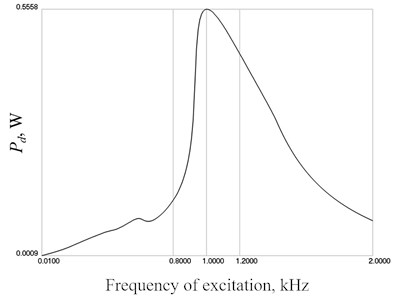a)${P}_{d}$ as the function of frequency of excitation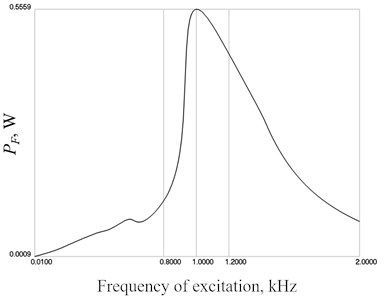b)${P}_{F}$ as the function of frequency of excitation

Theoretical calculation results show the dependence between frequency of excitation and average power consumption of the forces of dissipation Fig. 4(a) or average power consumption of the forces of excitation Fig. 4(b) as well. In both cases it was found that the highest dissipation forces and excitation forces was generated at the frequency of 1000 Hz. Also, when the frequency was increased up to 2000 Hz, the dissipation and excitation forces decreased. It happened that system came out of the ranges of resonance frequency and more energy was required to achieve the same result.

Average velocity as the function of frequency of excitation is presented in Fig. 5.

Fig. 5Average velocity as the function of frequency of excitation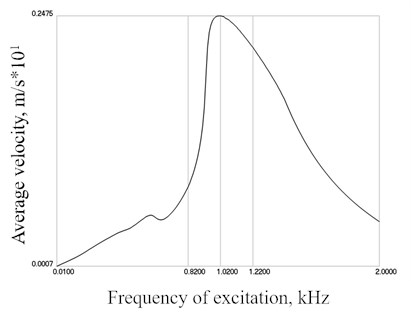Fig. 5 shows, that the highest velocity of surface cleaning was achieved when the excitation frequency was specified about 1020 Hz. Here, average cleaning velocity was determined to be about 0.02575 m/s. In other cases, when the oscillation frequency was increased or decreased, the average cleaning velocity was calculated to be lower. These theoretical calculation results revealed that it was necessary to choose the correct excitation frequency for the best surface cleaning result.

On the basis of the presented results acceptable dynamic regimes of the investigated vibrating system are chosen.

## 3. Experimental research of the influence of vibrations on cleaning of contaminated surfaces

Adhesion behavior of particles or particles and a surface has been studied for many years. Extensive reviews on particle adhesion mechanism have been reported [7-14]. Despite great efforts in scientific technology, the adhesion of particles and surfaces is a phenomenon that is far from being fully understood. This is due to many factors acting during the process, such as surface roughness and material type, and size, shape, electrostatic properties of particles as well.

Experimental research was performed with the purpose to approve the theory presented previously determining the influence of cleaning velocity of contaminated surface on vibration frequency as this dependence is directly related with adhesion forces between particles and the surface. Special device was designed and standard dust as a homogeneous layer was used.

### 3.1. Experimental methods used in research

Designed device which was used for experimental research is presented in Fig. 6. The main components of the mentioned device (Fig. 6) are those: mechanical system with rotational disc (Fig. 6(4)) and mounted gear with a plate of glass (Fig. 6(2, 3)); mechanical system with mounted piezoelectric cantilever (Fig. 6(1)); motion of mounted plate of glass was generated by varying forces of attached loads (Fig. 6(5)); force ${F}_{1}=$ const, and ${F}_{2}=$ (${F}_{1}÷$ (${F}_{1}+$ 0.02 N)); and inductive sensor (Fig. 6(6)). Mechanical system with rotational disc was made using two different discs. The one of them was made of steel (S235JR) with the diameter of 205 mm and thickness of 10 mm and was made immobile. The immobile disc was mounted on the antivibration table. Rotational disc was made of acrylic glass (poly-(methyl methacrylate) (PMMA) with the dimensions of 170 mm diameter and 5 mm thickness. This one was assembled with immobile disc through center holes with the bolt M14. Precise gear was made using 4 aluminium alloy pulleys with the dimensions of 25 mm diameter and 4 mm thickness. High resolution bearings were mounted inside the pulleys and fixed on two shafts fixed on rotational disc with the distance of 100 mm. A plate of glass (standardized microscope slide made of borosilicate glass was used for this purpose) was fixed on nylon wires mounted on these pulleys was mounted purposely for lower friction force. The ends of nylon wires were stretched by attached loads acting as balance weights. Dimensions of glass plate were decreased from standardized ones to 20 × 34 × 1 mm. Mechanical system with mounted piezoelectric cantilever was assembled separately from mechanical system with rotational disc. Therefore, in order to reduce the influence of vibrations this mechanical system with piezo cantilever was kept in right angle with glass plate in all experimental positions setting various angles between glass plate and initial horizontal position.

Piezo cantilever (produced by “Omega piezo”; model OPT 45/6.5/0.6) 1 was used as the source of vibrations with constant amplitude and varying frequency starting from 300 Hz up to 3000 Hz. Small layer of test object was placed on the glass base 2 with surface roughness of 0.001 μm. Glass base with the length of 34 mm was attached on two nylon threads stretched between four pulleys 3 mounted on the plastic rotatable disk 4 for the purpose to change angle between glass base and horizontal line. Acting forces 5 generated by loads were used for tension of nylon threads between the pulleys or creation glass plate motion. Inductive sensor 6 was used for specifying the distance between load and the surface of the sensor. Inductive sensor was connected with oscilloscope “PicoScope DAQ” for determination of the distance and time. These results were used for cleaning velocity calculations. During experiment the velocity of cleaning of contaminated surface was determined changing the frequency of piezo cantilever vibration.

Signal generator Agilent 3322OA and amplifier FLC Electronics F10A were used for excitation of vibrations in piezo cantilever. An exact amount of test object was obtained using precision weighing. A certain layer of test object with the dimensions of 5 × 20 × 1 mm was formed on glass surface. Piezo cantilever was approached near the test object since its adhesion force would not be able to move a glass base. Actuated vibrations created small impacts that start moving the particles of test object out of glass base.

Fig. 6Scheme of experimental setup: 1 – piezo cantilever, 2 – glass base, 3 – pulley, 4 – rotatable plastic disc, 5 – attached forces F1 and F2, 6 – inductive sensor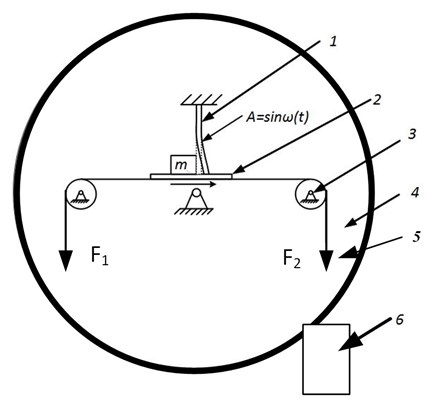Standard dust was used for experiments as the test object. The SE observation of the dust was carried out on ZEISS EVO MA10 scanning electron microscope (SEM) with an electron beam of 5 kV at room temperature. SEM analysis revealed heterogeneous microstructure of dust particles varying in size and shape (Fig. 7) corresponding the standard ISO 12103-1 Arizona Test Dust Contaminants, A4 Coarse Grade. However, the particles smaller than 0.97 μm were found Fig. 7(b). Chemical composition of standard dust is listed in Table 1. Our test dust is produced by “PTI Powder Technology Inc.”. Keeping in mind that the dust particles had different shape and size, and were rough as well, the adhesion forces between particles would be greater comparing with adhesion forces between the layer of dust and smooth glass base with the roughness of 0.001 μm. As the point of interest was adhesion between the surface and dust particles, thus the layer could be taken as a homogenous one as it was done in theoretical calculations.

Fig. 7SEM image of test dust Arizona A4: a) blocks found; b) small fragments on big ones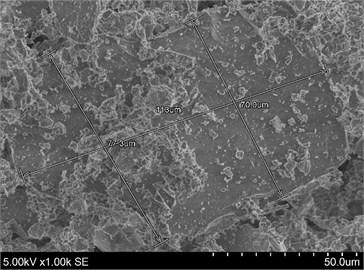a)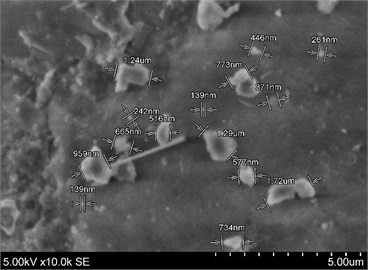b)

Table 1Typical chemical analysis of ISO 12103-1 Arizona test dust contaminant, A4 grade

 Element % of weight Element % of weight Silicon 69.0-77.0 Calcium 2.5-5.5 Aluminum 8.0-14.0 Magnesium 1.0-2.0 Iron 4.0-7.0 Titanium 0.0-1.0 Sodium 1.0-4.0 Potassium 2.0-5.0

### 3.2. Determination of cleaning velocity of contaminated surface

Test results were entered in software MATLAB R2016a version and calculation of the dependence of frequency on cleaning velocity of contaminated surface was performed. It was determined that the highest cleaning velocity was achieved when vibration frequency was about 1000 Hz (Fig. 8). Test results showed good agreement with theoretical calculation (Fig. 5).

Fig. 8Experimental research showing the influence of vibration frequency on cleaning velocity of contaminated surface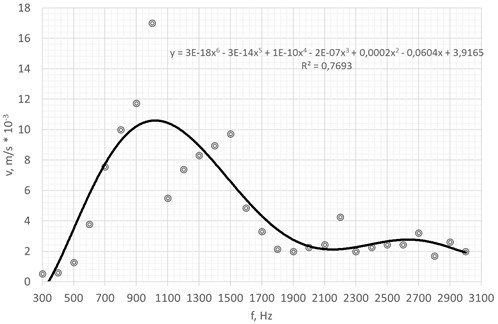However, during experimental research it was determined that the average cleaning velocity of test dust from the selected surface was about 30 % lower. These differences in cleaning velocities were determined by the following factors: air resistance, friction force, humidity, dust layer form conditions, different size of test dust particles, etc. These factors were not assessed during the theoretical calculation.

## 4. Conclusions

Vibrations of a device described by a nonlinear differential equation are investigated. Graphical relationships describing the motion in steady state regimes are obtained. Characteristics of motion as the functions of frequency of excitation are investigated. On the basis of the presented results the acceptable dynamic regimes are chosen for the investigated vibration based surface cleaning system.

Experimental research results approve theoretical calculation showing that the highest cleaning velocity can be obtained with the vibration frequency of approximately 1000 Hz. The proposed method of cleaning of contaminated surfaces can be used in automotive industry (for example for cleaning of air filters), in electronics (for purification of micro schemes), etc.

#### Cited by

Impacting Droplet Can Mitigate Dust from PDMS Micro-Post Array Surfaces
Improved numerical approximation of dry friction phenomena

05 December 2016
Accepted
01 May 2017
Published
15 May 2017
SUBJECTS
Mechanical vibrations and applications
Keywords
vibration
nonlinearity
characteristics of motion
surface cleaning
Author Contributions

Kazimieras Ragulskis contribution to this paper was the development of the theoretical background. Algimantas Bubulis contribution to this paper was the development of the experimental setup and analysis of experimental results. Darius Mažeika contribution to this paper was the development of the experimental setup. Rasa Kandrotaitė-Janutienė contribution to this paper was the development of the experimental setup and optical analysis of dust specimens. Liutauras Ragulskis contribution to this paper was theoretical calculation of velocities of excitation frequency. Audrius Bartkus contribution to this paper was the processing of experimental results.